﻿ NLVM 6 - 8 - Data Analysis & Probability Manipulatives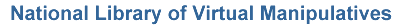Data Analysis & Probability (Grades 6 - 8)

Virtual manipulatives for Data Analysis & Probability, grades 6 - 8.Bar Chart – Create a bar chart showing quantities or percentages by labeling columns and clicking on values.Box Model – Randomly selects and displays draws from a box.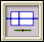Box Plot – Use this tool to summarize data using a box plot graph.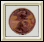Coin Tossing – Explore probability concepts by simulating repeated coin tosses.Hamlet Happens – Verify that rare events happen by drawing letters from a box.Histogram – Use this tool to summarize data using a histogram graph.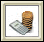Loan Calculator – Explore how to pay off a loan, and how interest affects payment.Pie Chart – Explore percentages and fractions using pie charts.Savings Calculator – Explore how savings, with or without regular deposits, grow over time.Scatterplot – Plot multiple data points in two dimensions and determine correlation.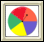Spinners – Work with spinners to learn about numbers and probabilities.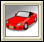Stick or Switch – Investigate probabilities of sticking with a decision, or switching.